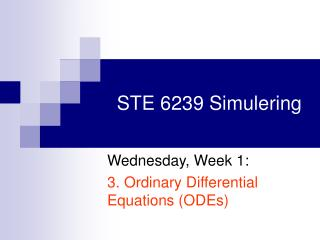Download PresentationSTE 6239 Simulering# STE 6239 Simulering - PowerPoint PPT Presentation

Download Presentation##### STE 6239 Simulering

Download Policy: Content on the Website is provided to you AS IS for your information and personal use and may not be sold / licensed / shared on other websites without getting consent from its author. While downloading, if for some reason you are not able to download a presentation, the publisher may have deleted the file from their server.

- - - - - - - - - - - - - - - - - - - - - - - - - - - E N D - - - - - - - - - - - - - - - - - - - - - - - - - - -
##### Presentation Transcript

1. STE 6239 Simulering Wednesday, Week 1: 3. Ordinary Differential Equations (ODEs)

2. 3.1. Preliminaries 3.1.1. Overview of ODE theory 3.1.2. The ordo-notation 3.1.3. The Taylor expansion of smooth functions, part I. 3.1.4. Change of variables in ODE (a material from the Geometrical Modelling course STE6238).

3. 3.2. Basic concepts and statements of error analysis • Numerical analysis • Order of approximation (= order of convergence) • Approximation error (= convergence error) • Rate of approximation (= rate of convergence) • Stability, general nonlinear problems • Condition number, general nonlinear problems • Stability, linear problems • Condition number, linear problems • Well-posed, ill-posed problem • Residual approximation error (= local approximation error) • Residual rate (= rate of residual approximation error) • The Lax Principle

4. 3.3. Example: numerical analysis of boundary problems for first order ODEs • The linear ODE of 1-st order can be solved analytically in closed form – see 3.1.1. • Standard numerical methods for non-linear ODEs of 1-st order present the simplest instance of approximate solution by finite-difference discretization, with the typical issues of numerical analysis. They are an object of study in the course on Numerical Methods SMN6191. • Revision/overviewof numerical methods for 1st order ODEs

5. 3.4. A comprehensive example: the 2nd order ODE mathematical model of stationary 1D heat conduction and gas diffusion 3.4.1. Modelling the physical phenomenon: • Determining the parameters • Derivation of the model 3.4.2 The resulting mathematical model 3.4.3. Scaling 3.4.4. Analysis of the model by numerical simulation: • FDM (the method of finite difference schemes) • FEM (the finite element method)

6. 3.5. The FDM approach 3.5.1. The FDM discretization paradigm • Discretization of the domain • Residual (local) approximation • Discretization of the equation • Discretization of the boundary conditions 3.5.2. Resulting discrete model • Computational complexity 3.5.3. Generalization: variable coefficients • The model and its discretization • Same structure and complexity

7. 3.6. The FEM approach 3.6.1. Discretization • The FEM paradigm • The Least Squares method • The Galerkin method • The test space • The boundary conditions 3.6.2.The Galerkin method for 2nd-order DE • Can use piecewise linear finite elements • Piecewise linear elements have only 1st derivative =>Least Squares method will not work with them. • Evaluation of the coefficients of the discretized system of equations • Result by FEM = Result by FDM Remark: see the discussion in the forthcoming material for Thursday, Week 1, on the comparison between FDM and FEM

8. 3.4. An example of a nonlinear model 3.4.1. An extension of the model in 3.4 to the non-linear case 3.4.2. An idea and algorithm for an iterative method based on the linear model 3.4 3.4.3. Discretization: the FDM approach • Discretization of the equation and boundary conditions & resulting iterative method • Numerical tests Remark: Step 3.4.3 can alternatively be carried through the FEM approach. However, FEM iterations are more expensive (i. e., have higher computational complexity)